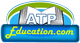ATP Education

# Mathematics NCERT Solutions for class 6 to 12 Hindi and English medium

MathematicsNCERT Solutions for Classes 6th to 12th in Hindi and English medium all chapters.

Mathematics NCERT Solutions for CBSE Board Classes 6 to 12, ncert solutions for all classes, Mathematics NCERT SOLUTIONS, Mathematics online NCERT solutions, Mathematics NCERT, Mathematics ncert, Mathematics ncert solutions, Mathematics ncert solutions for board exams, Mathematics ncert English book solutions, Mathematics book solutions, ncert Mathematics book solutions, Mathematics solved question answer for all exercise,

# NCERT Books Subject Mathematics for class class 6 to 12 in Hindi Medium

## Select Class for NCERT Books Solutions

#### NCERT Solutions